R语言逻辑回归和泊松回归模型对发生交通事故概率建模我们已经看到了如何考虑风险敞口，计算包含风险敞口的多个数量（经验均值和经验方差）的非参数估计量。让我们看看如果要对二项式变量建模。

• 未观察到该期间的索赔数量

• 索偿的数量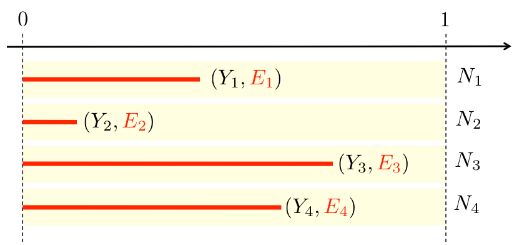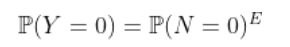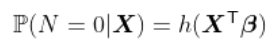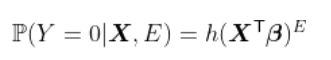> T1= contrat$nocontrat[I==FALSE]> nombre2 = data.frame(nocontrat=T1,nbre=0)> sinistres = merge(contrat,nombre)> sinistres$nonsin = (sinistres$nbre==0) 我们可以考虑的第一个模型基于标准的逻辑回归方法很好，但是很难用标准函数处理。尽管如此，始终有可能通过数值计算给定的最大似然估计量。 > optim(fn=logL,par=c(-0.0001,-.001),+ method="BFGS")$par 2.14420560 0.01040707$value 7604.073$countsfunction gradient42       10$convergence 0$messageNULL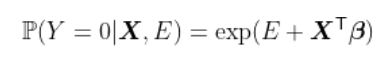Error: no valid set of coefficients has been found: please supply starting values

> reg=glm(nonsin~ageconducteur+offset(exposition),+ data=sinistresI,family=binomial(link="log"),+ control = glm.control(epsilon=1e-5,trace=TRUE,maxit=50),+ start=startglm,+ etastart=etaglm,mustart=muglm)Deviance = NaN Iterations - 1Error: no valid set of coefficients has been found: please supply starting values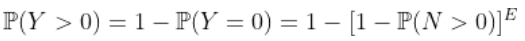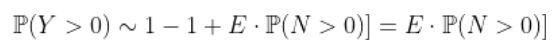> exp(coefficients(regreff))(Intercept)0.06776376

> 1-exp(param)/(1+exp(param)) 0.06747777

(Intercept)0.07279295

> lines(age,1-yml1,type="l",col="purple")> abline(h=exp(coefficients(regreff)),lty=2)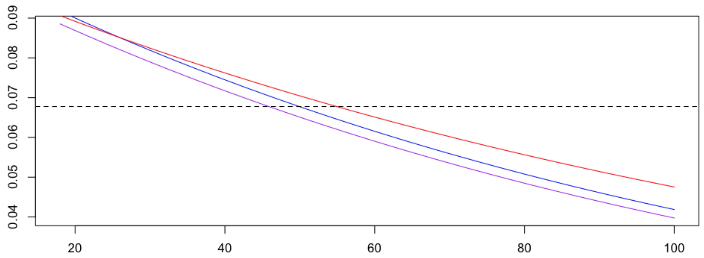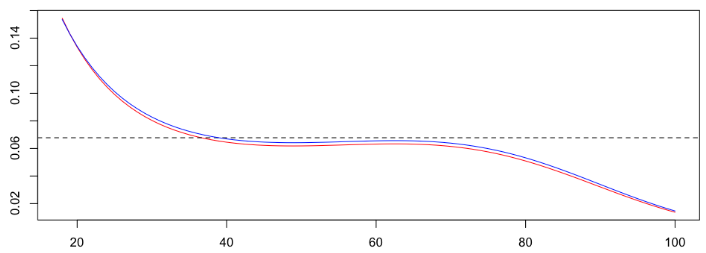https://wap.sciencenet.cn/blog-2674246-1243267.html

全部精选博文导读

GMT+8, 2022-1-19 08:29